NCERT Exemplar Class 11 Maths Chapter 5 Complex Numbers and Quadratic Equations are part of NCERT Exemplar Class 11 Maths. Here we have given NCERT Exemplar Class 11 Maths Chapter 5 Complex Numbers and Quadratic Equations.

## NCERT Exemplar Class 11 Maths Chapter 5 Complex Numbers and Quadratic Equations

Complex Numbers Class 11 Questions And Answers NCERT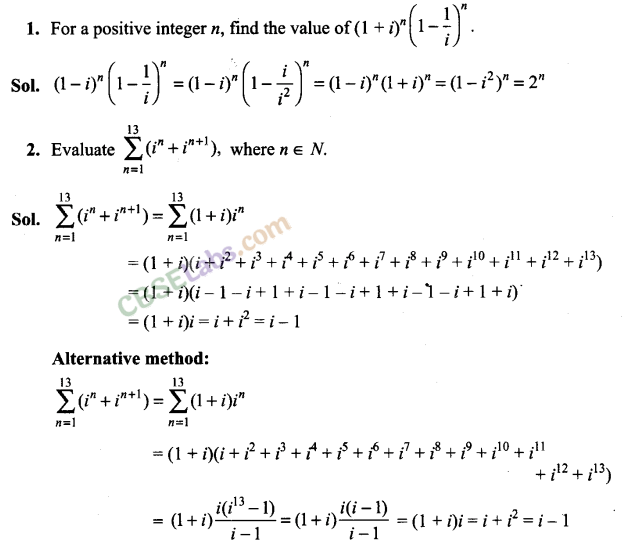NCERT Exemplar Class 11 Complex Numbers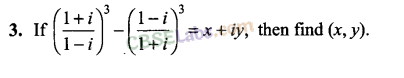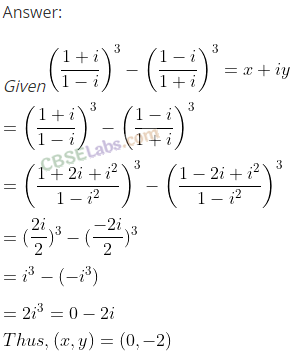Questions On Complex Numbers Class 11 NCERTNCERT Exemplar Class 11 Maths Ch 5

Q6. If a = cos θ + i sin θ, then find the value of (1+a/1-a)
Sol:  a = cos θ + i sin θQ10. Show that the complex number z, satisfying the condition arg lies on arg (z-1/z+1) = π/4 lies on a  circle.

Sol: Let z = x + iy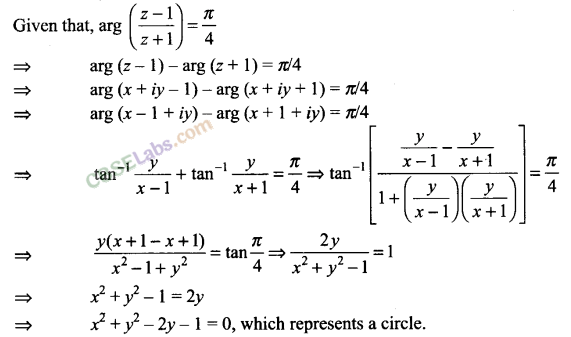Q11. Solve the equation |z| = z + 1 + 2i.
Sol: We have |z| = z + 1 + 2i
Putting z = x + iy, we get
|x + iy| = x + iy + 1+2i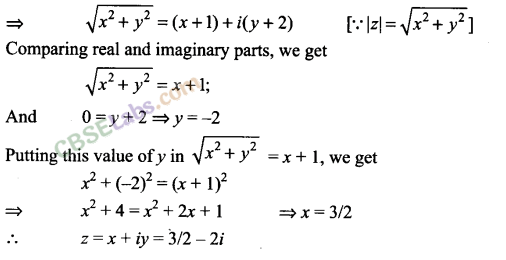Q12. If |z + 1| = z + 2( 1 + i), then find the value of z.
Sol: We have |z + 1
1 = z + 2(1+ i)
Putting z = x + iy, we get
Then, |x +
iy + 11 = x + iy + 2(1 + i)
|x + iy + l|=x + iy + 2(1 +i)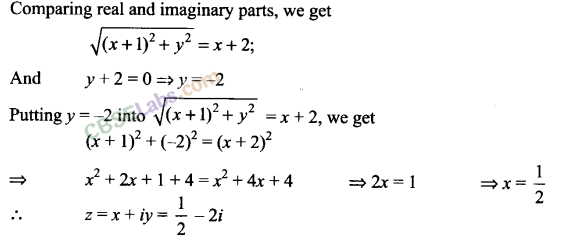Q13. If arg (z – 1) = arg (z + 3i), then find (x – 1) : y, where z = x + iy.
Sol: We have arg (z – 1) = arg (z + 3i), where z = x + iy
=>  arg (x + iy – 1) = arg (x + iy + 3i)
=> arg (x – 1 + iy) = arg [x + i(y + 3)]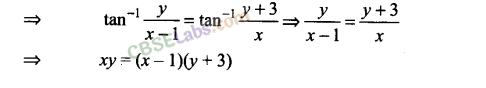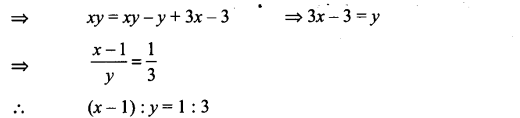Q14. Show that | z-2/z-3| = 2 represents a circle . Find its center and radius .
Sol:
We have | z-2/z-3| = 2
Puttingz=x + iy, we getQ15. If z-1/z+1 is a purely imaginary number (z ≠1), then find the value of |z|.

Sol: Let   z = x + iy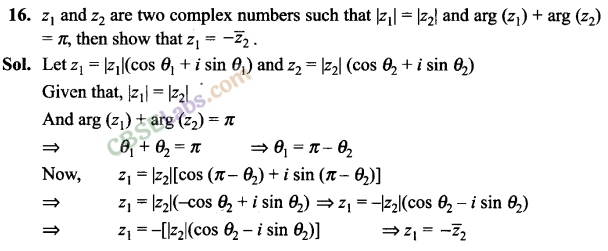Q17. If |z1 | = 1 (z1≠ -1) and z2 = z1 – 1/ z1 + 1 , then show that real part of z2 is zero .Q18. If Z1, Z2 and Z3, Z4 are two pairs of conjugate complex numbers, then find arg (Z1/ Z4) + arg (Z2/ Z3)
Sol. It is given that z1 and z2 are conjugate complex numbers.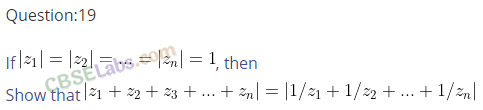Q20. If for complex number z1 and z2, arg (z1) – arg (z2) = 0, then show that |z1 – z2| = | z1|- |z2 |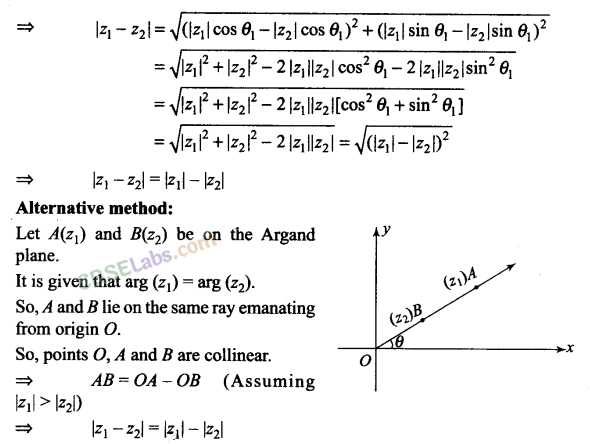Q21. Solve the system of equations Re (z2) = 0, |z| = 2.

Sol: Given that, Re(z2) = 0, |z| = 2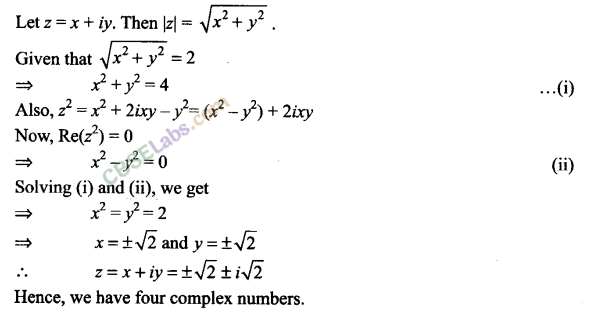Q22. Find the complex number satisfying the equation z + √2 |(z + 1)| + i = 0.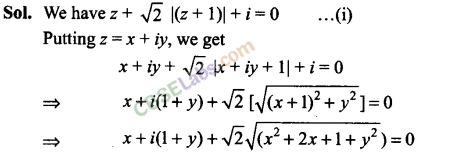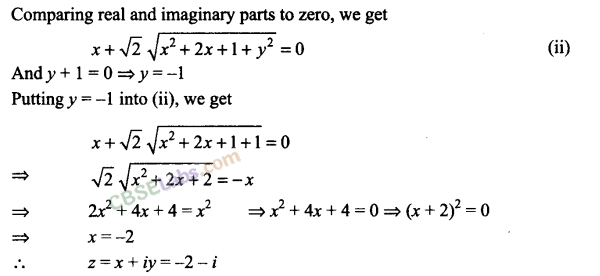Fill in the blanks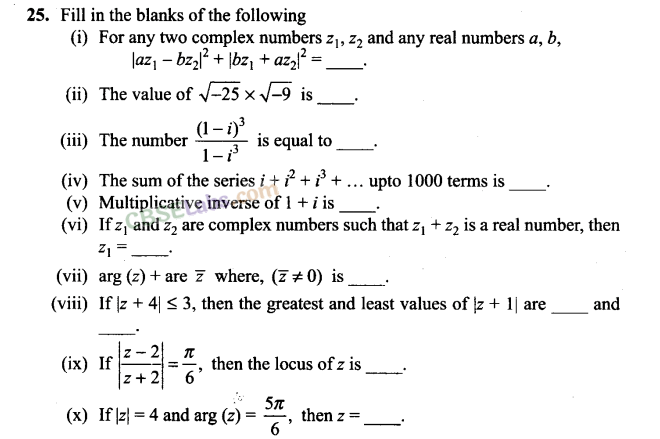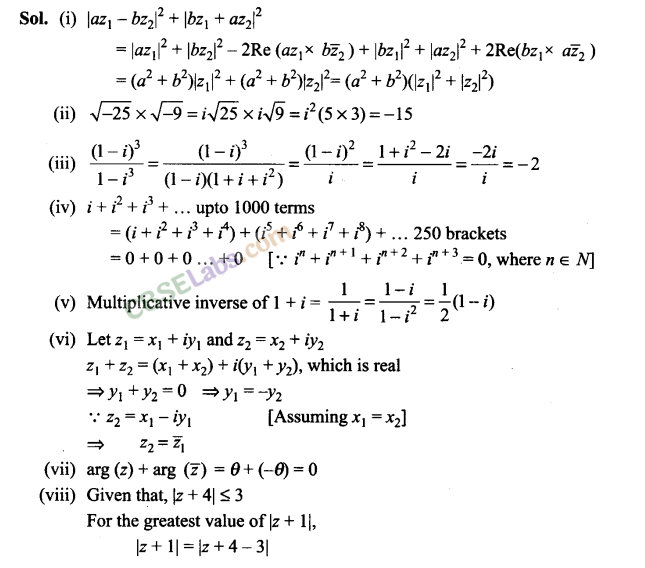True/False Type Questions

Q26. State true or false for the following.
(i) The order relation is defined on the set of complex numbers.
(ii) Multiplication of a non-zero complex number by -i rotates the point about origin through a right angle in the anti-clockwise direction.
(iii) For any complex number z, the minimum value of |z| + |z – 11 is 1.
(iv) The locus represented by |z — 11= |z — i| is a line perpendicular to the join of the points (1,0) and (0, 1).
(v) If z is a complex number such that z ≠ 0 and Re(z) = 0, then Im (z2) = 0.
(vi) The inequality |z – 4| < |z – 2| represents the region given by x > 3.
(vii) Let Z1 and Z2 be two complex numbers such that |z, + z2| = |z1 j + |z2|, then arg (z1 – z2) = 0.
(viii) 2 is not a complex number.

Sol:(i) False
We can compare two complex numbers when they are purely real. Otherwise comparison of complex numbers is not possible or has no meaning.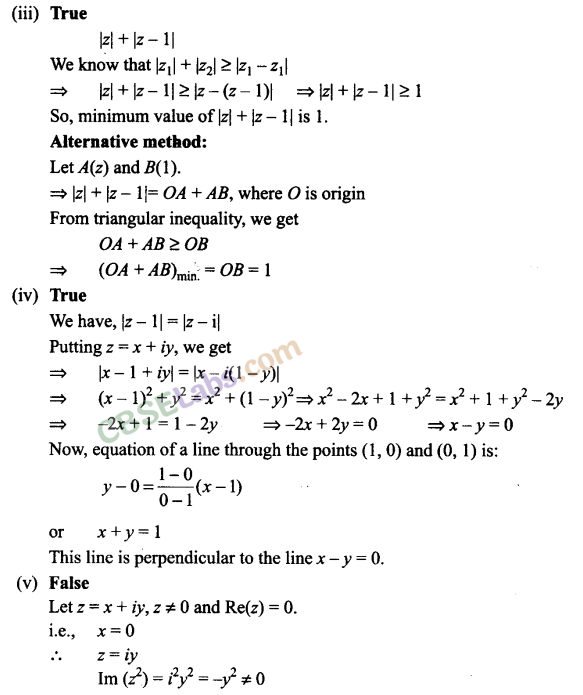Matching Column Type Questions
Q24. Match the statements of Column A and Column B.

 Column A Column B (a) The polar form of i + √3 is (i) Perpendicular bisector of segment joining (-2, 0) and (2,0) (b) The amplitude of- 1 + √-3 is (ii) On or outside the circle having centre at (0, -4) and radius 3. (c) It |z + 2| = |z – 2|, then locus of z is (iii) 2/3 (d) It |z + 2i| = |z – 2i|, then locus of z is (iv) Perpendicular bisector of segment joining (0, -2) and (0,2) (e) Region represented by |z + 4i| ≥ 3 is (v) 2(cos /6 +I sin /6) (0 Region represented by |z + 4| ≤ 3 is (Vi) On or inside the circle having centre (-4,0) and radius 3 units. (g) Conjugate of 1+2i/1-I  lies in (vii) First quadrant (h) Reciprocal of 1 – i lies in (viii) Third quadrant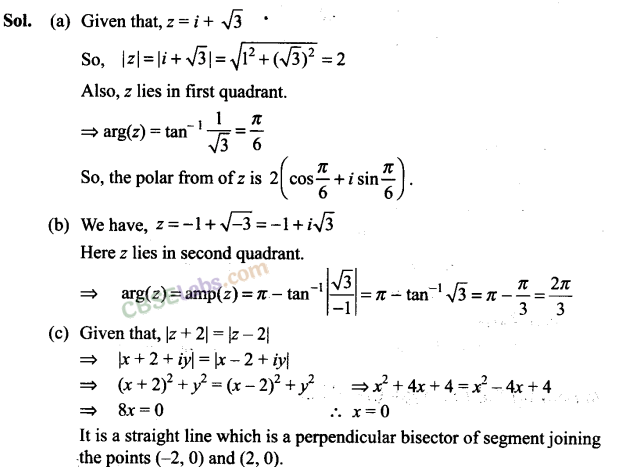Q28. What is the conjugate of 2-i / (1 – 2i)2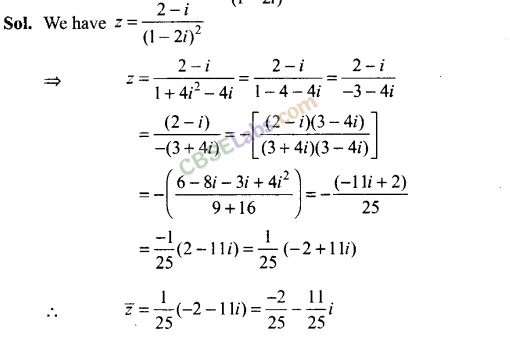Q29. If |Z1| = |Z2|, is it necessary that Z1 = Z2?
Sol: If |Z1| = |Z2| then z1 and z2 are at the same distance from origin.
But if arg(Z1) ≠arg(z2), then z1 and z2 are different.
So, if (z1| = |z2|, then it is not necessary that z1 = z2.
Consider Z1 = 3 + 4i and Z2 = 4 + 3i

Q30.If  (a2+1)2 / 2a –i = x + iy, then what is the value of x2 + y2?
Sol:
(a2+1)2 / 2a –i = x + iy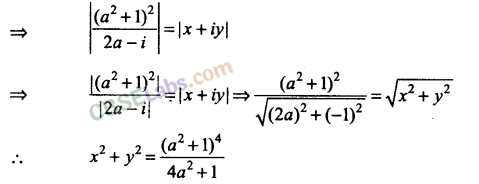Q31. Find the value of z, if |z| = 4 and arg (z) = 5π/6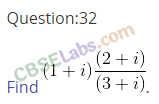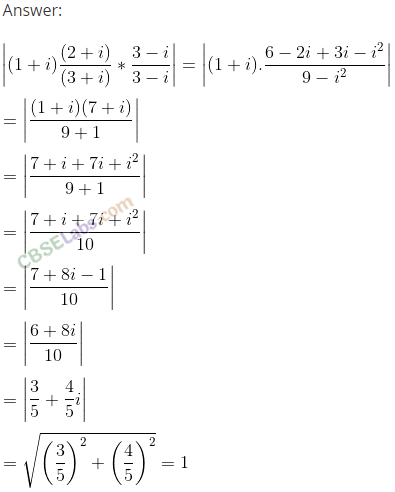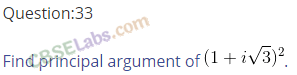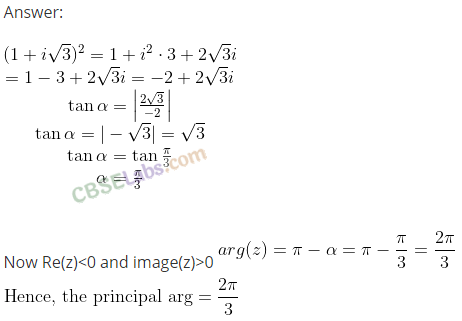Q34. Where does z lies, if | z – 5i / z + 5i  |  = 1?
Sol:
We have | z – 5i / z + 5i  |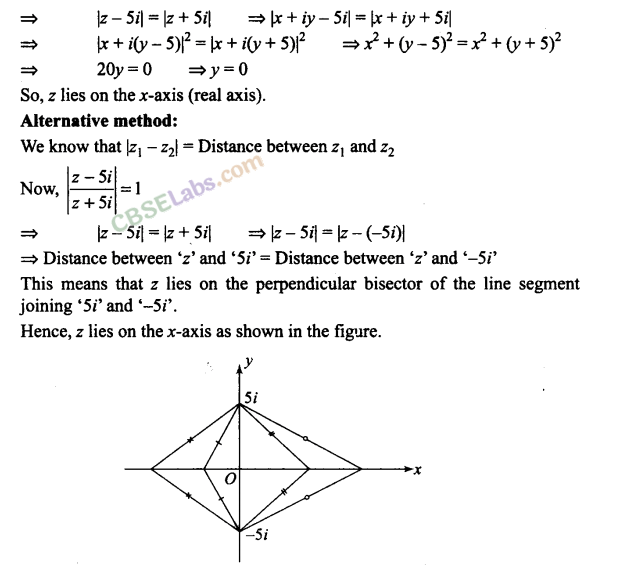Instruction for Exercises 35-40: Choose the correct answer from the given four options indicated against each of the Exercises.

Q35. sin x + i cos 2x and cos x – i sin 2x are conjugate to each other for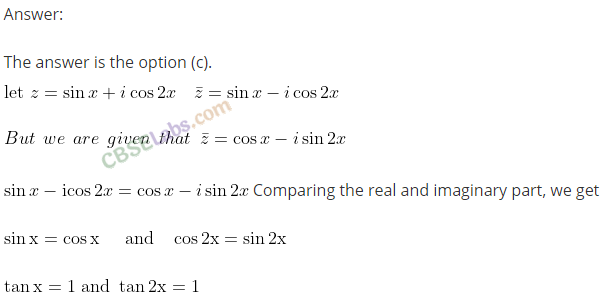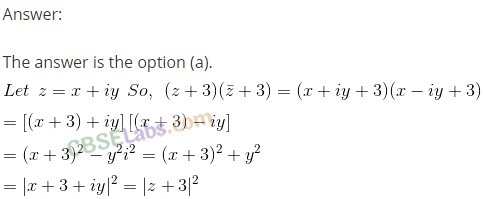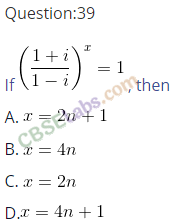Question 40.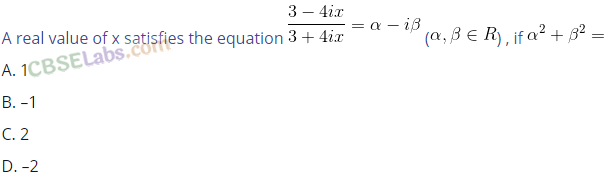Q41. Which of the following is correct for any two complex numbers z1 and z2?We hope the NCERT Exemplar Class 11 Maths Chapter 5 Complex Numbers and Quadratic Equations help you. If you have any query regarding NCERT Exemplar Class 11 Maths Chapter 5 Complex Numbers and Quadratic Equations, drop a comment below and we will get back to you at the earliest.